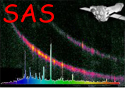XMM-Newton Science Analysis System

psfgen (psfgen-1.7) [xmmsas_20211130_0941-20.0.0]

# Parameters

This section documents the parameters recognized by this task (if any).

 Parameter Mand Type Default Constraints Event List Parameters image no string NULL valid file name A name of a fits file that was generated from xmmselect/evselect. No image is used if NULL. level no string ELLBETA valid arccuracy level value Accuracy level: LOW, MEDIUM, EXTENDED, HIGH, ELLBETA binning no int valid binning value On-chip-binning factor. Only works for certain camera mode coordtype yes string TEL valid coordinate value Coordinate Type: TEL (theta,phi), DET (detector coords), RAW (chip coords), POS (X,Y - sky coordinates, EQPOS (Ra, Dec), IMPIX (image pixel). The last three (POS,EQPOS,IMPIX) can only be used if an image is supplied. x no real The X coordinate of the centre of the PSF. If coordtypeTEL, then x is the off-axis angle (theta) which is expected in units of arcseconds. If coordtypeEQPOS then x is the right ascension in units of degrees. For other coordinate systems it should be entered in units of pixels. y no real The Y coordinate of the centre of the PSF. If coordtypeTEL, then y is the azimuthal angle (phi) which is expected in units of radians. If coordtypeEQPOS then y is the declination in units of degrees. For other coordinate systems it should be entered in units of pixels. ccdnr no int valid CCD number CCD number. This is mandatory if coordtype=RAW. energy no real list 3000.0Energy value list. Note that there is a minimum-maximum range weight no real list 1.0 Weighting to construct summation PSF from multiple PSFs with different energies. rotate no real 0.0Rotation angle of the PSF in units of degrees. If rotate is non-zero then the PSF will be rotated by this angle. The rotation is in the same direction as the position angle (PA). If rotate is not set but an image has been given via the image paremeter then the PSF will be rotated by the position angle (PA_PNT) stored in the image file header. If rotate is non-zero then it will override the value in the input image. xsize no int 199Width of the X-axis output image in pixels. ysize no int 199Width of the Y-axis output image in pixels. output no string psfimage.fits valid file name File name of the PSF image. Parameter Mand Type Default Constraints

XMM-Newton SOC -- 2021-11-30